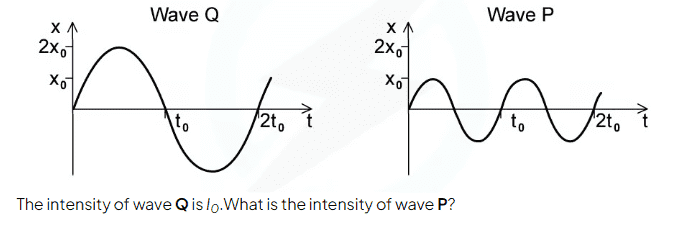# Intensity of a wave at half amplitude and twice frequency compared to a reference wave

• homeworkhelpls

#### homeworkhelpls

TL;DR Summary: How do i find the intensity of this wave?

I know I is proportional to amplitude / frequency squared, but I don't know what equation this comes from. And I don't know how to answer this.I know I is proportional to amplitude / frequency squared
Let's start with the basics: do you know the difference between amplitude and frequency?

Let's start with the basics: do you know the difference between amplitude and frequency?

What kind of wave is this? And what is x on the vertical axis? Is it linear displacement of the particles in the wave?

What kind of wave is this? And what is x on the vertical axis? Is it linear displacement of the particles in the wave?
doesnt matter, the question is used to ultimately prove that intensity of both waves is the same, its a proof question

Ok. So is intensity proportional to the square of the amplitude or of the frequency, or what?

Last edited:
@homeworkhelpls, you might be puzzled by these responses:
Let's start with the basics: do you know the difference between amplitude and frequency?
Ok. So is intensity proportional to the square of the amplitude or of the frequency, or what?
But I'd say the cause is some ambiguity in your post:
I know I is proportional to amplitude / frequency squared,
• ##(\frac{amplitude}{frequency})^2##
• either ##{amplitude}^2## or ##{frequency}^2##, but not sure which or whether it matters
• both ##{amplitude}^2## and ##{frequency}^2##
My guess is that you meant the third, whereas @Ibix read it as the second.

•Ibix
My guess is that you meant the third, whereas @Ibix read it as the second.
There's a point here about writing clearly, @homeworkhelpls . You saved yourself two characters by writing / writing of "and". You'd probably have had your answer this morning if you'd spent the two extra characters.

doesnt matter, the question is used to ultimately prove that intensity of both waves is the same, its a proof question
It does matter to know what quantities are represented on the axes. You may know it but out of context is not obvious.

It does matter to know what quantities are represented on the axes. You may know it but out of context is not obvious.
No i don't know it, you're not supposed to know it bro i saw the answer

•Motore
@homeworkhelpls, you might be puzzled by these responses:

But I'd say the cause is some ambiguity in your post:

• ##(\frac{amplitude}{frequency})^2##
• either ##{amplitude}^2## or ##{frequency}^2##, but not sure which or whether it matters
• both ##{amplitude}^2## and ##{frequency}^2##
My guess is that you meant the third, whereas @Ibix read it as the second.
Damn guys my bad lol, no all three guesses were wrong i meant the physics law of intensity where, I is directally proportional to amplitude squared

Maybe you will find this link useful.
lol, what if i have no heat source

•Motore
Damn guys my bad lol, no all three guesses were wrong i meant the physics law of intensity where, I is directally proportional to amplitude squared
See if this helps https://physics.info/intensity/

I know I is proportional to amplitude / frequency squared, but I don't know what equation this comes from. And I don't know how to answer this.
It is not clear what your difficulty is. You don’t need the equation to apply proportionality. For example: the area of a circle is 100cm²; the radius is halved; what is the new area? (No equations needed.)

From the graphs:

1: what is the value of ##\frac {A_P}{A_Q}## (where ##A## is amplitude)?

2: what is the value of ##\frac {f_P}{f_Q}## (where ##f## is frequency)?

In terms of proportionality:

3: how is intensity related to amplitude if all other parameters are kept constant?

4: how is intensity related to frequency, if all other parameters are kept constant?

If you can answer all 4 questions, you should be able to solve the problem.

No i don't know it, you're not supposed to know it bro i saw the answer
You know that this is related to some chapter in a book where a specific type of wave is described. Possibly just a plane wave in 1 dimension. But this is not the only wave possible and a linear dispalcement (position of particle) is not the only parameter used to describe a wave. What you know in the context of the specific book is not obvious for the people outside that context. A sound wave is most commonly described by the acoustic pressure and not particle displacement, for example.

Bruh relax I’ll just send answer
You know that this is related to some chapter in a book where a specific type of wave is described. Possibly just a plane wave in 1 dimension. But this is not the only wave possible and a linear dispalcement (position of particle) is not the only parameter used to describe a wave. What you know in the context of the specific book is not obvious for the people outside that context. A sound wave is most commonly described by the acoustic pressure and not particle displacement, for example

•Motore